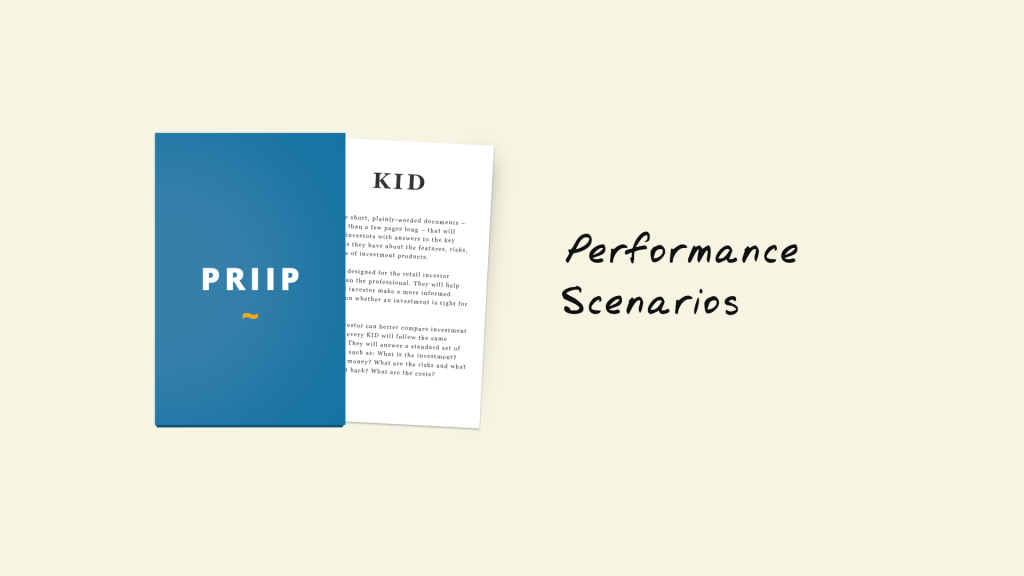As explained in our blogpost Update on KIDs for PRIIPs, a new scenario was introduced by the regulatory technical standards (RTS) dated April 2017. Besides the unfavourable, moderate and favorable scenarios it’s obligatory to show also the Stress-Scenario. In this article we will have a more detailed look into those calculations, which are all based on the Cornish-Fisher expansion, and will show how these scenarios expose a wide range of possible scenario returns.

Calculation of the scenarios for category 2 PRIIPs:

The unfavourable scenario shall be the value of the PRIIP at the 10th percentile. For the calculation of this scenario, the following formula is used:The moderate scenario, which represents the value of the PRIIP at the 50th percentile, is calculated as following:The last of the three scenarios which were already defined in the previous version of the RTS, the favourable one, is the value of the PRIIP at the 90th percentile. The formula of the scenario is:Beside the number of trading periods in the recommended holding period (), these formulas include the mean, the volatility, the skewness as well as the kurtosisandwhich can be measured from the return distribution.

For the calculation of the new Stress-Scenario, it is necessary to identify a sub interval of length w which corresponds to the following intervals:

 1 year > 1 year Daily prices 21 63 Weekly prices 8 16 Monthly prices 6 12

The next step is, to identify for each sub interval of length w the historical lognormal returns, where, which are used to calculate the volatility based on the formula below starting fromrolling until:Whereis the count of number of observations in the sub interval andis the mean of all the historical lognormal returns in the corresponding sub interval. After that, it’s necessary to find the value that corresponds to the 99th percentile for 1 year and the 90th percentile for the other holding periods, which is called the stressed volatility.

Withfor a holding period of 1 year andfor the other holding periods now it’s possible to calculate the value of the Stress-Scenario with the following formula: